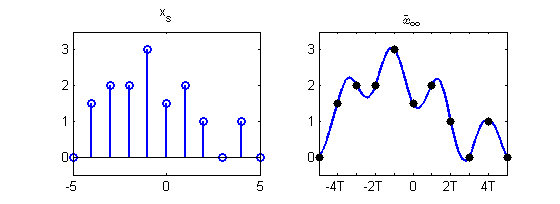Skip to main content

# 10.4: Perfect Reconstruction

•• Contributed by Richard Baraniuk et al.
• Victor E. Cameron Professor (Electrical and Computer Engineering) at Rice University

## Introduction

If certain additional assumptions about the original signal and sampling rate hold, then the original signal can be recovered exactly from its samples using a particularly important type of filter. More specifically, it will be shown that if a bandlimited signal is sampled at a rate greater than twice its bandlimit, the Whittaker-Shannon reconstruction formula perfectly reconstructs the original signal. This formula makes use of the ideal lowpass filter, which is related to the sinc function. This is extremely useful, as sampled versions of continuous time signals can be filtered using discrete time signal processing, often in a computer. The results may then be reconstructed to produce the same continuous time output as some desired continuous time system.

## Perfect Reconstruction

In order to understand the conditions for perfect reconstruction and the filter it employs, consider the following. As a beginning, a sufficient condition under which perfect reconstruction is possible will be discussed. Subsequently, the filter and process used for perfect reconstruction will be detailed.

Recall that the sampled version $$x_s$$ of a continuous time signal $$x$$ with sampling period $$T_s$$ has a spectrum given by

$X_{s}(\omega)=\frac{1}{T_{s}} \sum_{k=-\infty}^{\infty} X\left(\frac{\omega-2 \pi k}{T_{s}}\right).$

As before, note that if $$x$$ is bandlimited to $$(−\pi /T_s, \pi /T_s)$$, meaning that $$X$$ is only nonzero on $$(−\pi /T_s, \pi /T_s)$$, then each period of $$X_s$$ has the same form as $$X$$. Thus, we can identify the original spectrum $$X$$ from the spectrum of the samples $$X_s$$ and, by extension, the original signal $$x$$ from its samples $$x_s$$ at rate $$T_s$$ if $$x$$ is bandlimited to $$(−\pi /T_s, \pi /T_s)$$.

If a signal $$x$$ is bandlimited to $$(−B,B)$$, then it is also bandlimited to $$(−\pi /T_s, \pi /T_s)$$ provided that $$T_s< \pi /B$$. Thus, if we ensure that $$x$$ is sampled to $$x_s$$ with sufficiently high sampling angular frequency $$\omega_s=2 \pi /T_s>2B$$ and have a way of identifying the unique $$(−\pi /T_s, \pi /T_s)$$ bandlimited signal corresponding to a discrete time signal at sampling period $$T_s$$, then $$x_s$$ can be used to reconstruct $$\tilde{x}=x$$ exactly. The frequency $$2B$$ is known as the angular Nyquist rate. Therefore, the condition that the sampling rate $$\omega_s=2 \pi /T_s>2B$$ be greater than the Nyquist rate is a sufficient condition for perfect reconstruction to be possible.

The correct filter must also be known in order to perform perfect reconstruction. The ideal lowpass filter defined by $$G(\omega)=T_s(u(\omega+\pi/T_s)−u(\omega−\pi/T_s))$$, which is shown in Figure $$\PageIndex{1}$$, removes all signal content not in the frequency range $$(−\pi /T_s, \pi /T_s)$$. Therefore, application of this filter to the impulse train $$\sum_{n=-\infty}^{\infty} x_{s}(n) \delta\left(t-n T_{s}\right)$$ results in an output bandlimited to $$(−\pi /T_s, \pi /T_s)$$.

We now only need to confirm that the impulse response $$g$$ of the filter $$G$$ satisfies our sufficient condition to be a reconstruction filter. The inverse Fourier transform of $$G(\omega)$$ is

$g(t)=\operatorname{sinc}\left(t / T_{s}\right)=\left\{\begin{array}{cc} 1 & t=0 \\ \frac{\sin \left(\pi t / T_{s}\right)}{\pi t / T_{s}} & t \neq 0 \end{array}\right. ,$

which is shown in Figure $$\PageIndex{6}$$. Hence,

$g\left(n T_{s}\right)=\operatorname{sinc}(n)=\left\{\begin{array}{cc} 1 & n=0 \\ \frac{\sin (\pi n)}{\pi n} & n \neq 0 \end{array}=\left\{\begin{array}{ll} 1 & n=0 \\ 0 & n \neq 0 \end{array}=\delta(n)\right.\right. .$

Therefore, the ideal lowpass filter $$G$$ is a valid reconstruction filter. Since it is a valid reconstruction filter and always produces an output that is bandlimited to $$(−\pi /T_s, \pi /T_s)$$, this filter always produces the unique $$(−\pi /T_s, \pi /T_s)$$ bandlimited signal that samples to a given discrete time sequence at sampling period $$T_s$$ when the impulse train $$\sum_{n=-\infty}^{\infty} x_{s}(n) \delta\left(t-n T_{s}\right)$$ is input.

Therefore, we can always reconstruct any $$(−\pi /T_s, \pi /T_s)$$ bandlimited signal from its samples at sampling period $$T_s$$ by the formula

$x(t)=\sum_{n=-\infty}^{\infty} x_{s}(n) \operatorname{sinc}\left(t / T_{s}-n\right).$

This perfect reconstruction formula is known as the Whittaker-Shannon interpolation formula and is sometimes also called the cardinal series. In fact, the sinc function is the infinite order cardinal basis spline $$\eta_{\infty}$$. Consequently, the set $$\left\{\operatorname{sinc}\left(t / T_{s}-n\right) \: | \: n \in \mathbb{Z}\right\}$$ forms a basis for the vector space of $$(−\pi /T_s, \pi /T_s)$$ bandlimited signals where the signal samples provide the corresponding coefficients. It is a simple exercise to show that this basis is, in fact, an orthogonal basis.Figure $$\PageIndex{1}$$: The above plots show the ideal lowpass filter and its inverse Fourier transform, the sinc function.Figure $$\PageIndex{2}$$: The plots show an example discrete time signal and its Whittaker-Shannon sinc reconstruction.

## Perfect Reconstruction Summary

This module has shown that bandlimited continuous time signals can be reconstructed exactly from their samples provided that the sampling rate exceeds the Nyquist rate, which is twice the bandlimit. The Whittaker-Shannon reconstruction formula computes this perfect reconstruction using an ideal lowpass filter, with the resulting signal being a sum of shifted sinc functions that are scaled by the sample values. Sampling below the Nyquist rate can lead to aliasing which makes the original signal irrecoverable as is described in the subsequent module. The ability to perfectly reconstruct bandlimited signals has important practical implications for the processing of continuous time signals using the tools of discrete time signal processing.